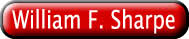Mutual Fund Performance Measures, Factor Models, and Fund Style and Selection William F. Sharpe www-sharpe.stanford.edu www-leland.stanford.edu/~wfsharpe

 Mutual Fund Performance Measures Use statistics from: historic frequency distribution many periods Example: combination of mean and standard deviation for past 36 months To predict statistics for: future probability distribution one period Example: combination of mean and standard deviation for next month

 Decisions One Fund One Fund plus borrowing or lending One fund from a given asset class or category A portfolio of potentially many funds

 Portfolio Theory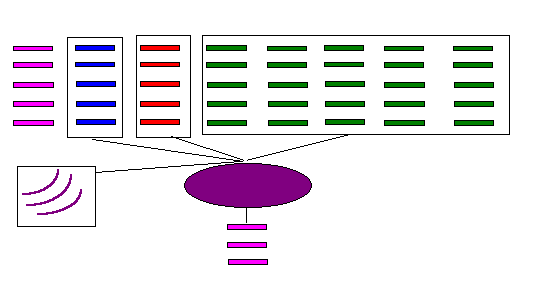Hierarchic Taxonomic Procedures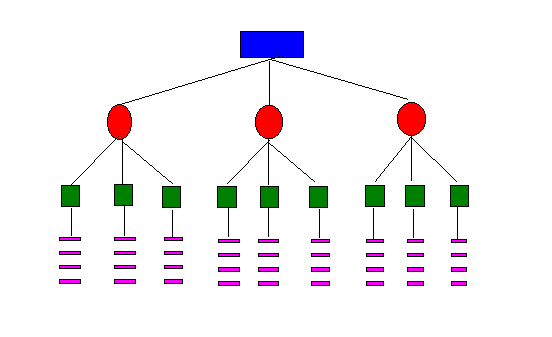Statistics: M Ex Ante: Expected Return Expected geometric return etc. Ex Post: Arithmetic average return Geometric average return Compounded total return over period etc.

 Statistics: S Ex Ante: Standard Deviation of Return Variance of Return Expected loss etc. Ex Post: Standard deviation of return Variance of Return Average loss etc.

 Performance Measures Return M Utility-based M - k * S Scale-independent M / S

 Variables Total Return Fund Return Excess Return Fund Return - Return on a risk-free instrument Differential Return Fund Return - Return on an appropriate benchmark portfolio

 Absolute and Relative Measures Absolute Use statistics as computed for all funds Relative Each fund assigned to a peer group Performance of funds ranked within each peer group Comparisons based on: Differences Ratios Rankings Stars 5 stars: top 10% 4 stars: next 22.5% etc.

Frequently-used Measures

Relative

 Total Return Excess Return Differential Return Return Lipper Utility-based Morningstar (form) Scale-independent Morningstar (subst.) Micropal

Absolute

 Total Return Excess Return Differential Return Return selection mean (alpha) Utility-based Scale-independent Sharpe ratio selection Sharpe ratio

 Scale-independent Measures Variable = Return on A minus return on B Strategy requires zero investment long position in A short position in B Change in value can be doubled by doubling sizes of positions For scale k: Mk = k* M1 SDk = k* SD1 Mk / SDk = M1 / SD1 Therefore, ratio is scale-independent

 Scale-independent Measures with Positive Expected Returns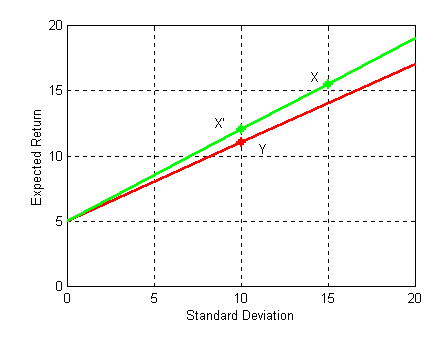Scale-independent Measures with Negative Average Returns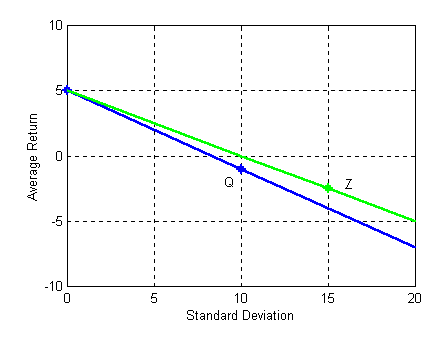Inappropriateness of Total Return M/S Measures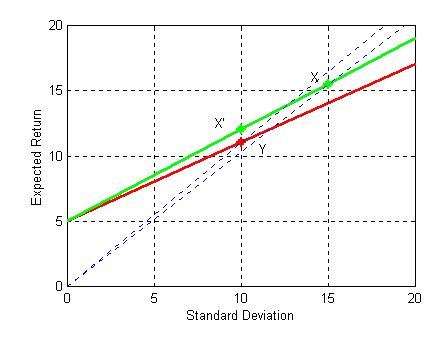Morningstar Peer Groups Peer Groups Asset classes Categories Asset Classes Domestic equity International equity Taxable bond Municipal bond Domestic equity categories Diversified (9) Specialty (9) Hybrid Convertible

Morningstar Diversified Equity Categories

Based on portfolio composition

• price/earnings, price/book
• market capitalization

Averaged over past three years

Style Boxes

 Large Value Large Blend Large Growth Medium Value Medium Blend Medium Growth Small Value Small Blend Small Growth

Morningstar Ratings

Stars:

• Rank within asset class (e.g. equity)
• 3-year, 5 year, 10 year and weighted average of 3,5, and 10 year

Category Ratings:

• Rank within asset category (e.g. Large Growth equity)
• 3-year
• Load charges not taken into account

Percentages:

 1 (worst) 2 3 4 5 (best) 10% 22.5% 35% 22.5% 10%

 Morningstar Statistics, 3-year Ratings M Compounded return on fund - compounded return on Treasury bills Loss if fund return > Treasury bill return, loss = 0 if fund return < Treasury bill return, loss = - (fund return - bill return) S Average Monthly Loss sum ( monthly loss) takes all 36 months into account

 Average Monthly Loss versus Standard Deviation of Monthly Returns, Morningstar Diversified Equity Funds, 1994-1996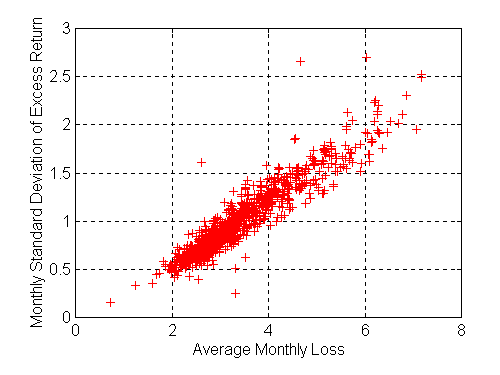Average Monthly loss versus function of Monthly Mean and Std. Deviation Morningstar Diversified Equity Funds, 1994-1996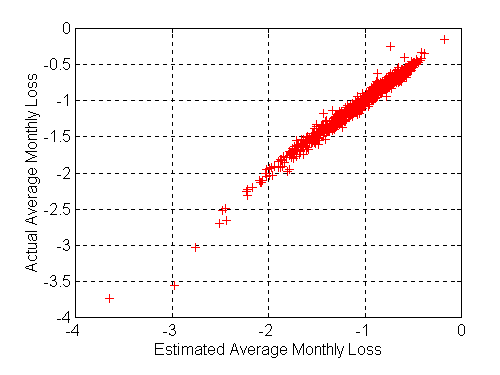Morningstar Risk-adjusted Rating RARf = Mf / M_ - Sf / S_ M_ if mean ( Mf ) >= compound return on Treasury bills, mean ( Mf ) if mean ( Mf ) < compound return on Treasury bills, compound return on Treasury bills S_ mean ( AMLf )

 Morningstar Risk-adjusted Ratings as Utility-based Measures RARf = Mf / M_ - Sf / S_ = ( 1/M_ ) * [ Mf - ( M_ / S_ ) * Sf ] Rankings unaffected by initial constant ( 1/M_ ) Rankings depend on: Mf - k * Sf where: k = M_ / S_

 A bi-linear VnM Utility Function with threshold = 4% and utility ratio = 2.5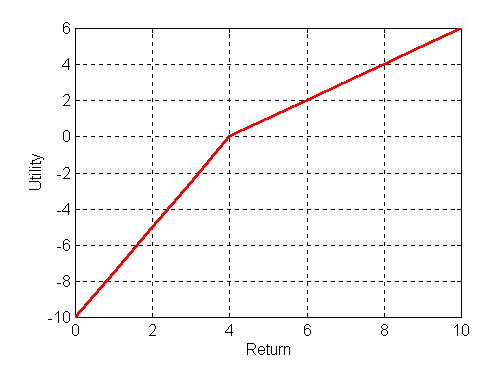Optimal Leverage when Utility = Return - k*Risk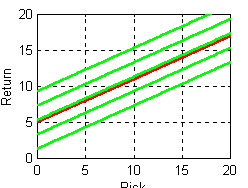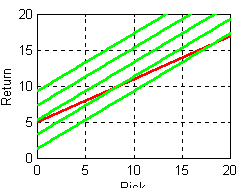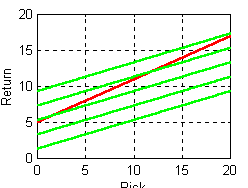Optimal Leverage when Utility = Return - k*Risk2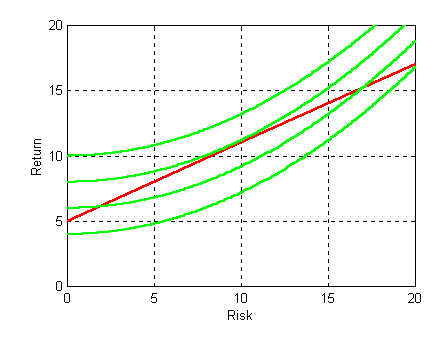Indifference Curves and Iso-M/S lines: k = M_ / S_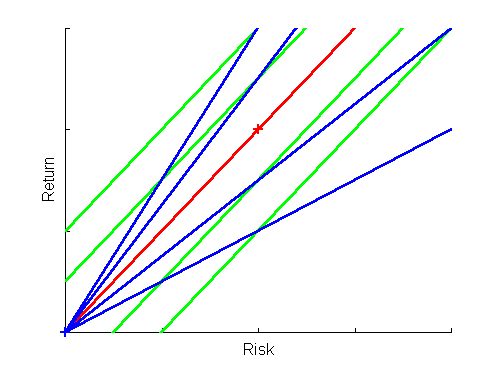Indifference Curves and Iso-M/S lines: k > M_ / S_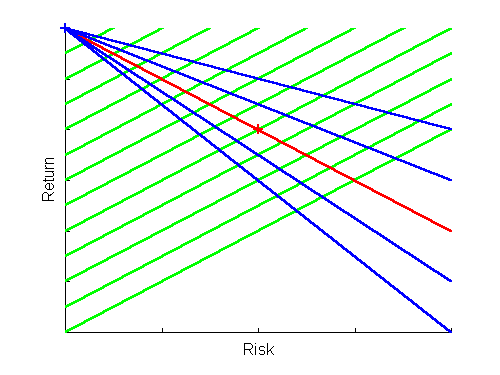Sharpe Ratio Ranks versus Category Rankings, Morningstar Diversified Equity Funds, 1994-1996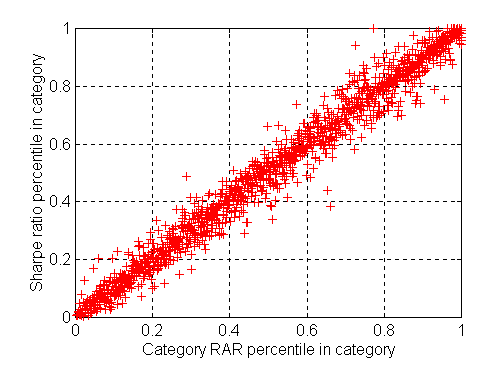Three-year Star Ratings and Mean-variance combinations, Morningstar Diversified Equity Funds, 1994-1996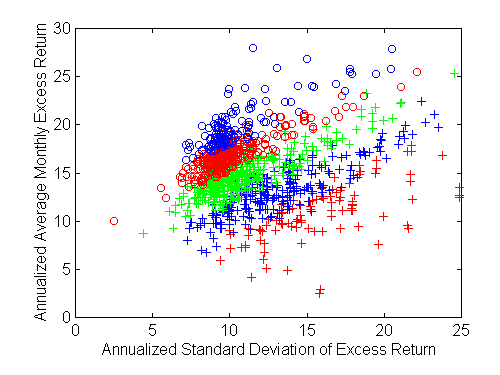An Asset Class Factor Model

R~f = [ b1f F~1 + b2f F~2 + ... + bnf F~n ] + e~f

 R~f Fund return F~1 ,...,F~n Asset class returns b1f ,..., bnf Fund asset class exposures (style) : sum = 1 [ ... ] Fund style return e~f Fund selection return: e~f i uncorrelated with e~f j

Benchmark Portfolios and Asset Exposures

R~f = [ b1f F~1 + b2f F~2 + ... + bnf F~n ] + e~f

 R~f Fund return F~1 ,...,F~n Asset class returns b1f ,..., bnf Benchmark portfolio composition [ ... ] Benchmark portfolio return e~f Fund differential return

Methods for Selecting a Benchmark

 Historic Average Current Projected Composition MStar Category MStar Style Regression Actual Returns Retrospective Returns Style Analysis Actual Returns Retrospective Returns Projection FER Proposal

 Taxonomic Factor Models All conditions for a general asset class factor model hold plus For any given fund f One bif = 1 All other bif's = 0 Fund expected return = asset class expected return + fund alpha Fund Variance = asset class variance + fund selection variance

 Overall Portfolio Return R~p = [ b1p F~1 + b2p F~2 + ... + bnp F~n ] + e~p where: bjp = X1 b1j + X2 b2j + ... + Xn bnj e~p = X1 e~1 + X2 e~2 + ... + Xn e~m [...] = (style) return on assets ( R~A ) e~p = selection return

Selection Return Statistics

Ex post

 mean ( e~f ) Average selection return ( alpha ) stddev ( e~f ) Selection return variability

Ex ante

 expected ( e~f ) Expected selection return ( alpha ) stddev ( e~f ) Selection return risk

 Factor-model Based Analysis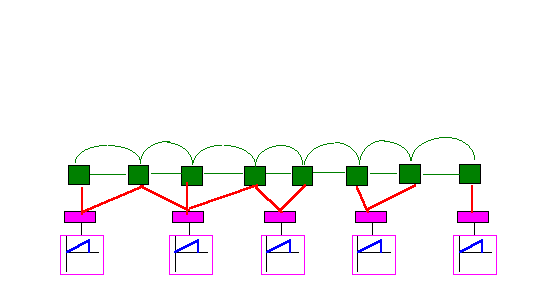Factor-model Based Analysis: Optimization Inputs Asset Classes Expected Returns Standard Deviations Correlations Funds Styles ( Benchmark portfolios) Expected selection returns (alphas) Selection risks Investor Risk tolerance: t other constraints, assets, liabilities, etc

 Optimization with Unlimited Short Positions in Assets Creating a hedge fund Long: fund Short: fund's benchmark asset mix Zero investment required Return is scale-independent Asset allocation unaffected by scale of investment Select Xi to maximize: Xi expected (ei ) - ( Xi 2 Var ( ei ) ) / t

 Optimal Position in a Fund with Unlimited Short Positions in Assets Xi = [ expected (ei ) / Var ( ei ) ) ] * ( t / 2 ) Amount of risk taken: Xi * stdev ( ei ) = [ expected (ei ) / stdev ( ei ) ] * ( t / 2 ) = [ selection Sharpe ratio ] * ( t / 2 ) Relative values independent of investor preferences

 Choosing a Fund for an Asset Class Position with a Taxonomic Factor Model Assume asset allocation is fixed Then: Ep = EA + X1 expected ( e1 ) + . . . + Xm expected ( em ) Vp = VA + X12 variance ( e1 ) + .... + Xm2 variance ( em ) Utility: [ EA - VA / t ] + [ X1 expected ( e1 ) - X12 variance ( e1 ) / t ] + . . . + [ Xm expected ( em ) - Xm2 variance ( em ) / t ]

 The Optimal Fund for an Asset Class with a Taxonomic Factor Model Xj is a given constant From the funds in the asset class, select the fund for which [ Xj expected ( ef ) - Xj2 variance ( ef ) / t ] is the largest Equivalently, select the fund with the largest value of: expected ( ef ) - ( Xj / t ) * variance ( ef ) A utility-based differential return measure with k a function of: the amount to be invested in the asset class ( Xj ) the investor's risk tolerance (t)

 The Optimal Fund for a Small Portion of a Portfolio The preferred fund for an investment of Xj in asset class j has maximum: z = expected ( ef ) - ( Xj / t ) * variance ( ef ) If Xj is small: ( Xj / t ) * variance ( ef ) is small z is approximately equal to expected ( ef ) = alpha Hence best fund is the one with the largest alpha relative to an appropriate benchmark

Correlations of Percentiles within Categories

 SR Cat. Star Alpha SSR Sharpe Ratio 1.000 0.986 0.945 0.831 0.744 Category Rating 0.986 1.000 0.957 0.829 0.735 Star Rating 0.945 0.957 1.000 0.790 0.694 Selection Mean (Alpha) 0.831 0.829 0.790 1.000 0.940 Selection Sharpe Ratio 0.744 0.735 0.694 0.940 1.000

 Style Analysis Alpha Ranks versus Category Rankings, Morningstar Diversified Equity Funds, 1994-1996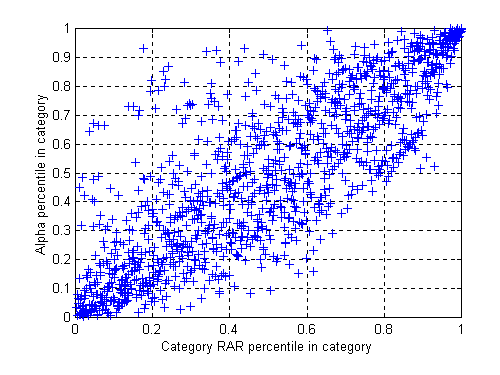Style Analysis Selection Sharpe Ratios versus Category Rankings, Morningstar Diversified Equity Funds, 1994-1996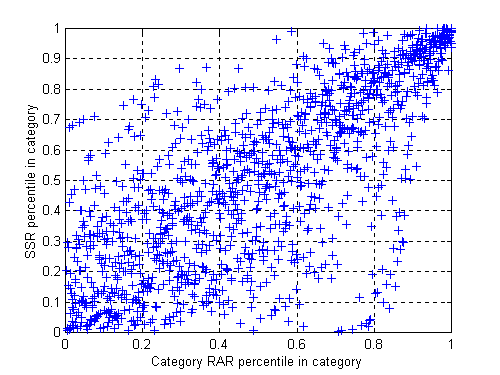Conclusions (1) Hierarchic taxonomic approaches will generally be suboptimal lower-level characteristics not taken into account when making decisions asset category characteristics not taken into account when allocating among asset classes fund characteristics not taken into account when allocating among asset classes and categories No universal single measure can provide a sufficient statistic for choosing one fund in each category, or multiple funds in each category

 Conclusions (2) Need good estimates of: future asset exposures appropriate benchmark portfolio (fund style) future fund selection risk future fund selection expected return This information should be combined optimally with estimates of future asset risks, expected returns and correlations investor risk tolerance and other characteristics All useful predictors of future performance should be taken into account include fund expense ratios, turnover, etc..